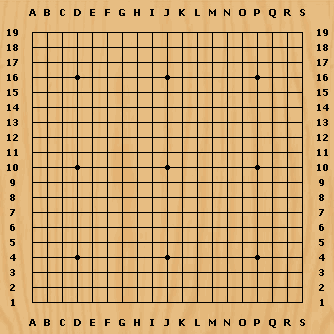Fun with FactorialsA while back my youngest son, who is an avid Go player, asked me, “Is it true there are more possible Go games than there are atoms in the universe?”“Absolutely,” I replied, “Let’s fire up FileMaker Pro and prove it.” (I wasn’t about to let a rare teachable moment slip by.) “If memory serves, the number of atoms in the universe is estimated be roughly 10^80. Since a Go board is 19 by 19, in theory there are 361 possible first moves (19^2), followed by 360 possible second moves for each of those 361 first moves, followed by 359 possible third moves for each of the 360 possible second moves, and so on. Therefore the total number of moves can be calculated as 361 x 360 x 359… x 1, or, more simply, 361! (i.e., 361 factorial).” Continue reading “Fun with Factorials”